Computers are incredibly good at math. That's why the invention of computers took place! Mathematicians and physicians invested hours calculating results from their formulas and data. Now, a computer can "compute" results for them in a snap of a finger. Moreover, in the earlier days, there used to be specific programming languages that majorly supported mathematical operations. FORTRAN is one of those examples. Still, with time and maturity of programming languages, mathematical calculations have become an integral part of every programming language, and JavaScript also is not an exception from the same. In this article, we will cover the details of the Math object in JavaScript, which helps the user to perform all kinds of mathematical operations.

• What is the Math object in JavaScript?
• Constants provided by Math object.
• Methods provided by Math Object.

## What is the Math object in JavaScript?

JavaScript's Math object performs mathematical operations on numbers. Unlike other global objects, Math is not a constructor. In other words, all the properties and methods of Math are static, and one can call them by using Math as an object without creating it. Additionally, the Math object provides a set of properties and methods for mathematical constants and functions. If we are using an IDE/Editor such as "Visual Code," we can check the properties and methods provided by the Math object by using the dot(.) operator with the "Math" object as shown below: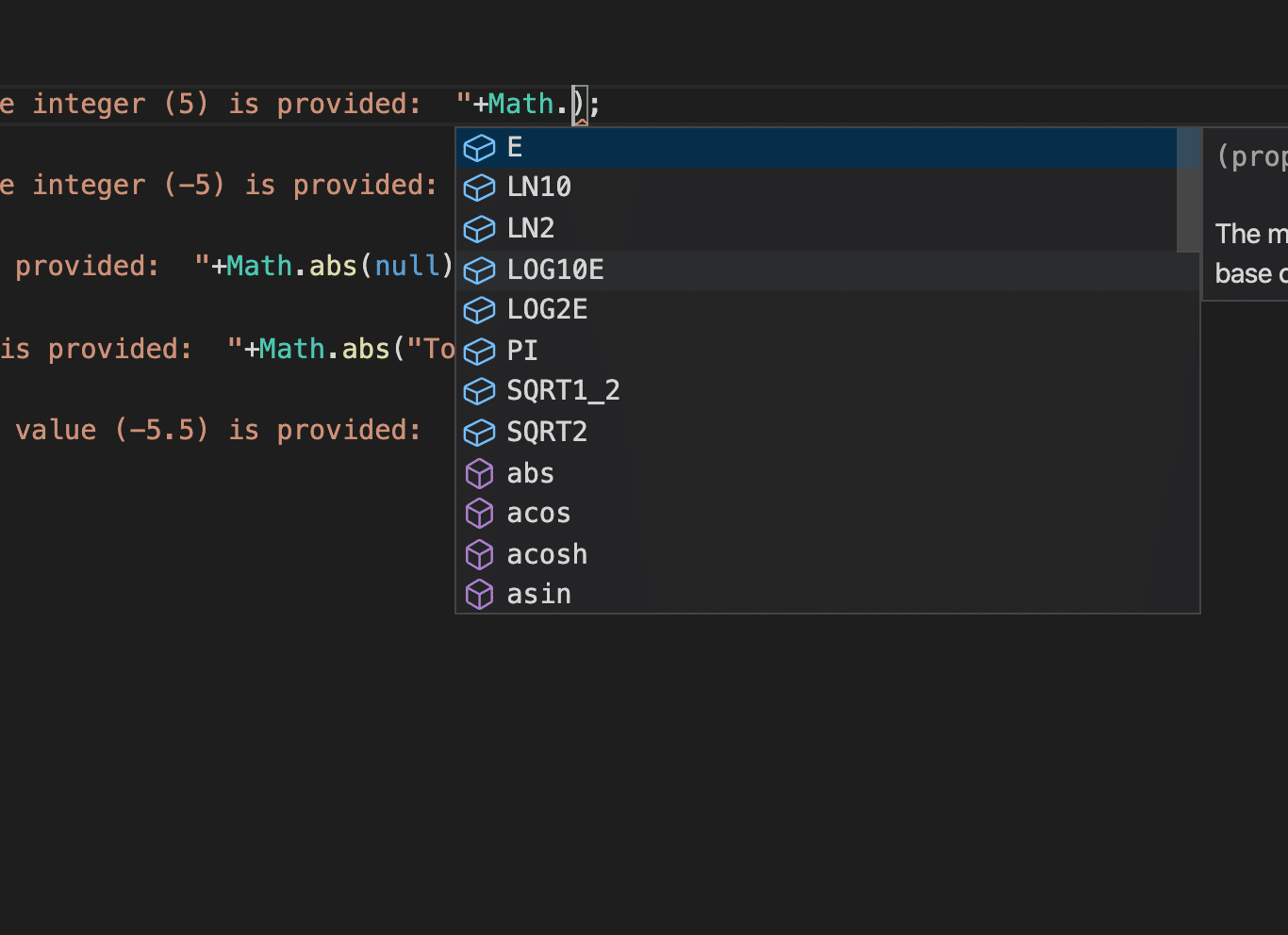As the above image shows, when we start scripting with the help of Visual Studio Code, and we type "Math." the VS code IntelliSense provides a list of methods and variables available in Math Object.

Let's discuss all these properties and methods provided by the Math object in detail in the below sections:

### Constants provided by Math Object

Constants are available in almost all programming languages. They are nothing but variables which can be assigned a value only once and can't change again during the scope of the program. Assume you need to use a value of PI, i.e., 3.1416, in your programming in multiple places. If you start using the actual value, then there might be a chance where it can get missed or value change. In another case, if you need to change the value from 3.1416 to 3.142 to then, you need to change everywhere.

Another way to handle this is to create a variable and assign the value, use this variable in the program, but there is a chance where you can reassign it. There is a concept of constants, which helps to prevent this. Whereby, a programmer can assign value to a variable only once. Moreover, if a programmer tries to reassign a new value, the compiler will throw an error.

Now to ease it further, the Math object in JavaScript already contains certain variables such as PI, E, LN2, etc. and as the programmer can't change the values of these variables. These are called constants provided by the Math object.

The JavaScript Math object provides following constants which solve various day to day mathematical problems:

• PI: Returns PI value that is 3.141592653589793

• SQRT2: Returns value of the square root of 2 that is 1.4142135623730951

• SQRT1_2: Returns value of the square root of ½ that is 0.7071067811865476

• LOG10E: *Returns value of Log of e to the base 10 that is 0.4342944819032518

• E: Returns value of Euler's number that is 2.718281828459045

• LN2: Returns value of Logarithmic value of 2 that is 0.6931471805599453

• LN10: Returns value of Logarithmic value of 10 that is 2.302585092994046

• LOG2E: Returns value of Log of e to the base 2 that is 1.4426950408889634

Let's see the usage of all these constants with the help of following code snippet:

``````<html>

<body>

Demonstrating Math constants in javascript.

</br>

<script type = "text/javascript">

document.write("Value of PI is: "+Math.PI);

document.write("</br>");

document.write("Value of Square root of 2 is: "+Math.SQRT2);

document.write("</br>");

document.write("Value of Square root of 1/2 is: "+Math.SQRT1_2);

document.write("</br>");

document.write("Value of Log of E to the base 10 is: "+Math.LOG10E);

document.write("</br>");

document.write("Value of Log of E to the base 2 is: "+Math.LOG2E);

document.write("</br>");

document.write("Value of Logarithm value of 2 is: "+Math.LN2);

document.write("</br>");

document.write("Value of Logarithm value of 10 is: "+Math.LN10);

document.write("</br>");

document.write("Value of Logarithm value of E is: "+Math.E);

document.write("</br>");

</script>

</body>

</html>

``````

Save the file with the name mathConstants.html. After that, open it in any browser (Chrome, Firefox, or IE). It should show the output as: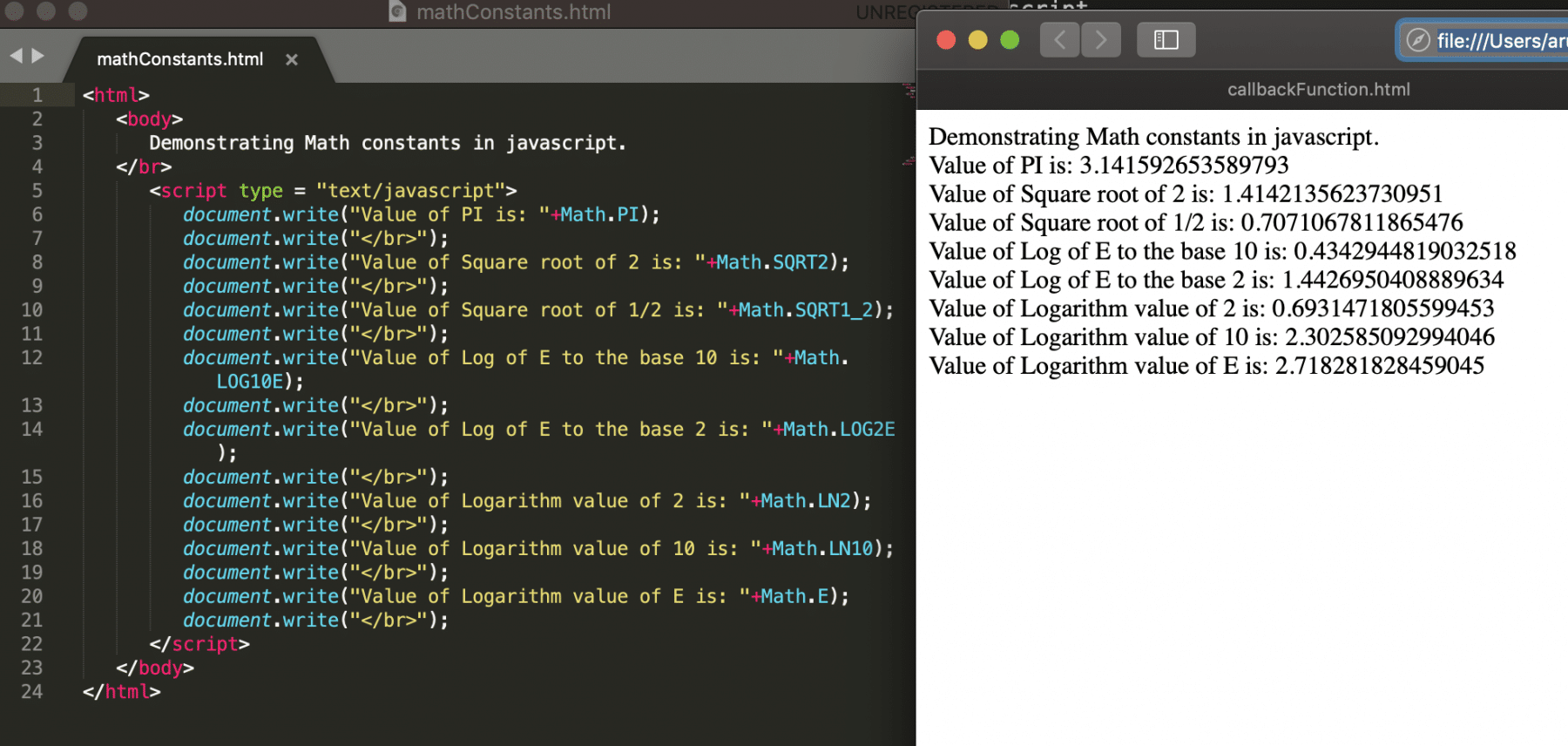### Methods provided by Math object

The Math object provides various methods also, which can perform mathematical calculations. Few of them are:

#### Abs()

This method will return the absolute value of a given number. Moreover, its syntax looks like below:

Syntax:

``````var variableName = 123;
Math.abs(variableName);
``````

Let's understand it further for various variable types with the help of following code snippet:

``````<html>

<body>

Demonstrating Math abs() method in javascript.

</br>

<script type = "text/javascript">

var breakLine = "</br>";

document.write("Absolute value if positive integer (5) is provided:  "+Math.abs(5));

document.write(breakLine);

document.write("Absolute value if negative integer (-5) is provided:  "+Math.abs(-5));

document.write(breakLine);

document.write("Absolute value if null is provided:  "+Math.abs(null));

document.write(breakLine);

document.write("Absolute value if string is provided:  "+Math.abs("Tools QA"));

document.write(breakLine);

document.write("Absolute value if decimal value (-5.5) is provided:  "+Math.abs(-5.5));

document.write(breakLine);

</script>

</body>

</html>

``````

Save the file with name absoluteMethods.html. After that, open it in any browser (Chrome, Firefox, or IE). It should show the output as: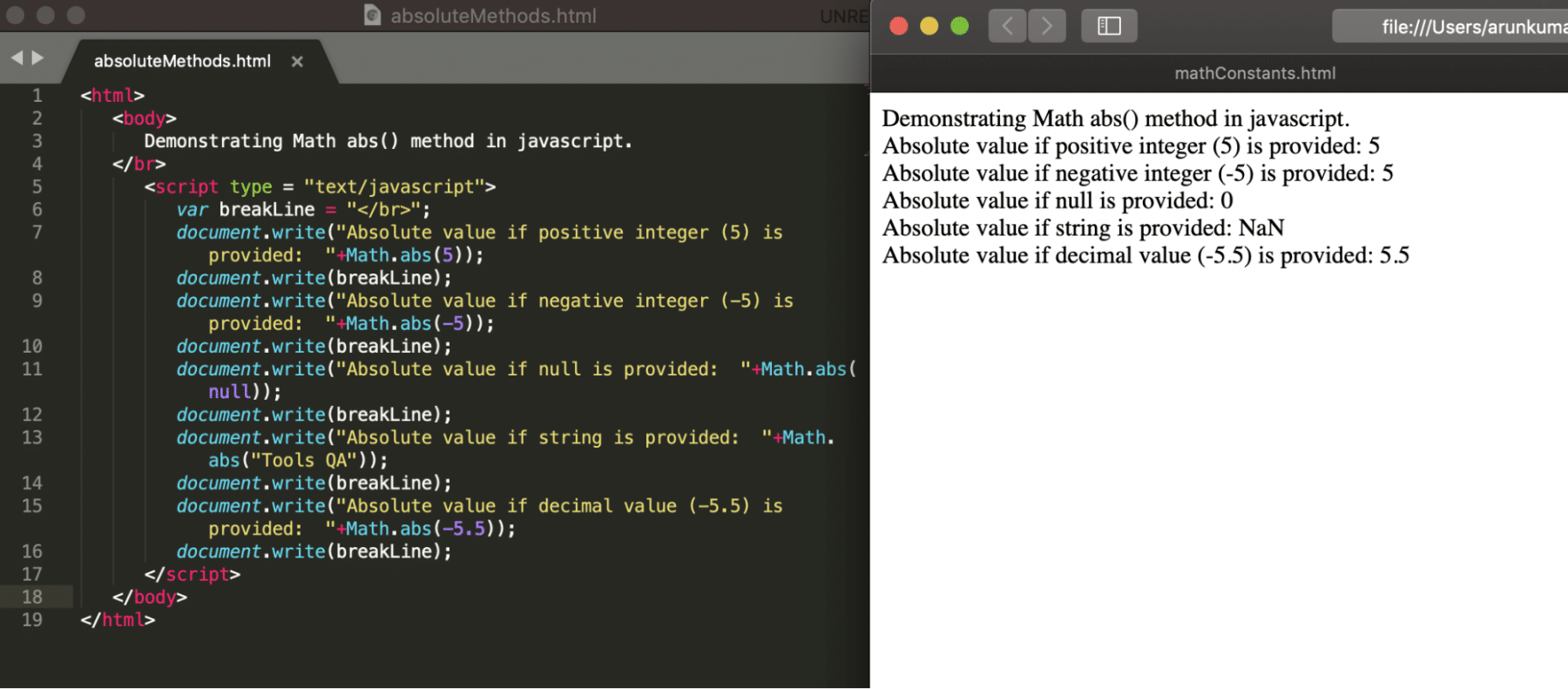#### acos()

The acos() method will return the cosine value between 0 to value of PI (i.e., 3.141592653589793). The acos is the arccosine(in radians) of the given number. As we know, in mathematics, arccosine is an inverse of cosine.

Note: input can be a value between -1 to 1.

Additionally, its syntax looks like below:

Syntax:

``````var radians = -1;
``````

Let's understand the usage of acos() for various variable types with the help of following code snippet:

``````<html>

<body>

Demonstrating Math acos() method in javascript.

</br>

<script type = "text/javascript">

var breakLine = "</br>";

document.write("Arccosine value if positive integer (5) is provided:  "+Math.acos(1));

document.write(breakLine);

document.write("Arccosine value if negative integer (-5) is provided:  "+Math.acos(-1));

document.write(breakLine);

document.write("Arccosine value if zero integer (0) is provided:  "+Math.acos(0));

document.write(breakLine);

document.write("Arccosine value if null is provided:  "+Math.acos(null));

document.write(breakLine);

document.write("Arccosine value if string is provided:  "+Math.acos("Tools QA"));

document.write(breakLine);

</script>

</body>

</html>

``````

Save the file with name arccosineMethod.html. After that, open it in any browser (Chrome, Firefox, or IE). It should show the output as: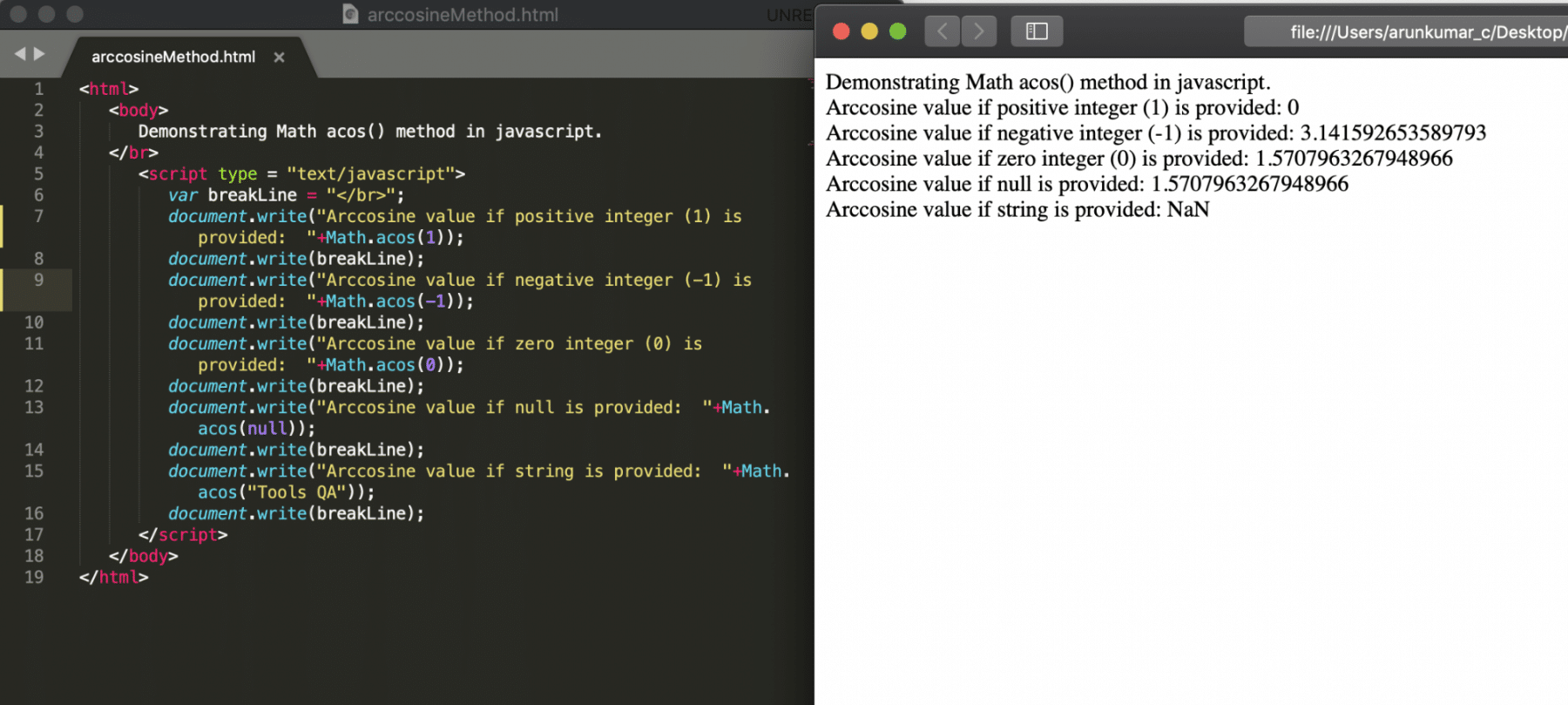#### acosh()

This function calculates the hyperbolic arc cosine value for the provided input. Another name of the acosh() is the "inverse hyperbolic cosine" function. Moreover, its syntax looks like below:

Syntax:

``````var radians = 5;

``````

Let's understand the usage of acosh() for various variable types with the help of following code snippet:

``````<html>

<body>

Demonstrating Math acosh() method in javascript.

</br>

<script type = "text/javascript">

var breakLine = "</br>";

document.write("Arccosine value if positive integer (20) is provided:  "+Math.acosh(20));

document.write(breakLine);

document.write("Arccosine value if negative integer (-20) is provided:  "+Math.acosh(-20));

document.write(breakLine);

document.write("Arccosine value if zero integer (0) is provided:  "+Math.acosh(0));

document.write(breakLine);

document.write("Arccosine value if null is provided:  "+Math.acosh(null));

document.write(breakLine);

document.write("Arccosine value if string is provided:  "+Math.acosh("Tools QA"));

document.write(breakLine);

</script>

</body>

</html>
``````

Save the file with name hyperbolicArccosineMethod.html. After that, open it in any browser (Chrome, Firefox, or IE). It should show the output as: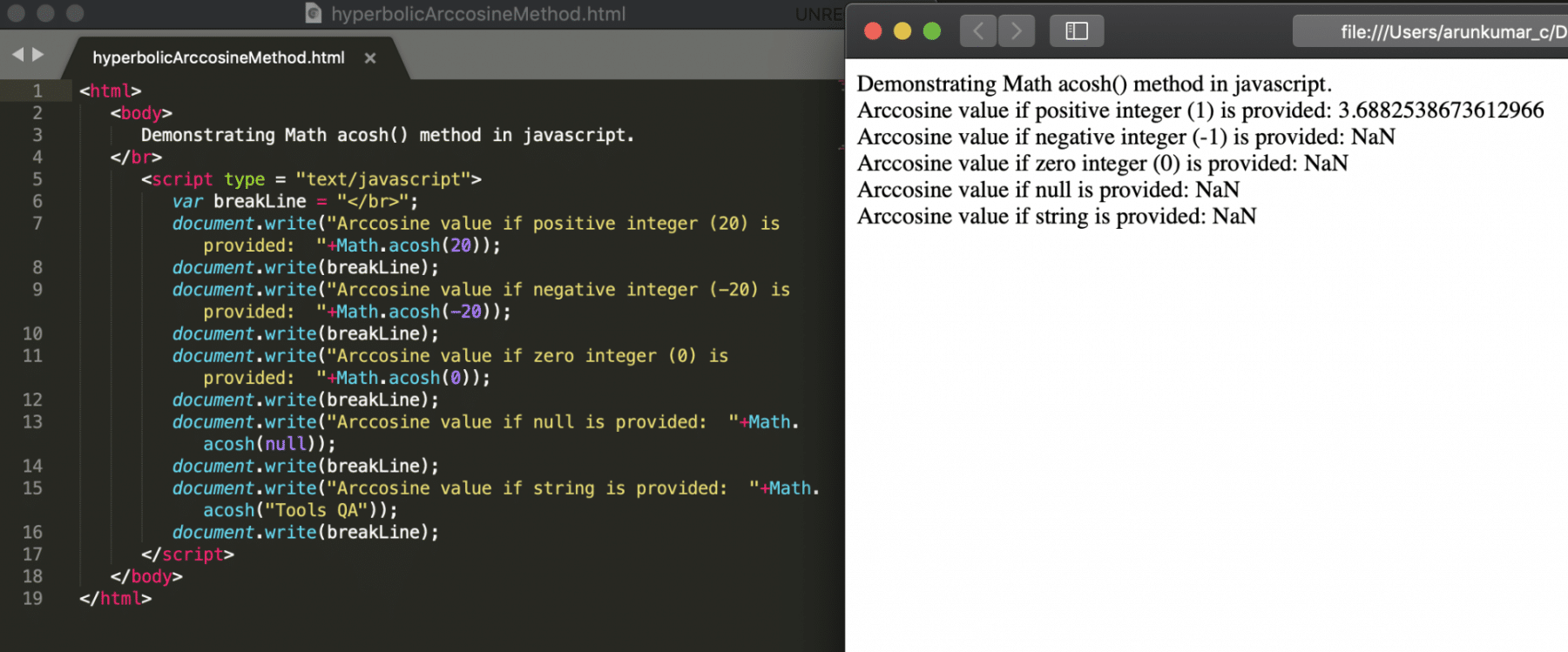#### asin()

The asin() method will return the sine value between -PI/2 to  PI/2. The asin is the arcsine(in radians) of the given number. As we know, in mathematics, arcsine is an inverse of sine.

Note: input can be a value between -1 to 1.

Additionally, its syntax looks like below:

Syntax:

``````var radians = -1;

``````

Let's understand the usage of asin() for various variable types with the help of following code snippet:

``````<html>

<body>

Demonstrating Math asin() method in javascript.

</br>

<script type = "text/javascript">

var breakLine = "</br>";

document.write("Arcsine value if positive integer (1) is provided:  "+Math.asin(1));

document.write(breakLine);

document.write("Arcsine value if negative integer (-1) is provided:  "+Math.asin(-1));

document.write(breakLine);

document.write("Arcsine value if zero integer (0) is provided:  "+Math.asin(0));

document.write(breakLine);

document.write("Arcsine value if null is provided:  "+Math.asin(null));

document.write(breakLine);

document.write("Arcsine value if string is provided:  "+Math.asin("Tools QA"));

document.write(breakLine);

</script>

</body>

</html>

``````

Save the file with name arcsineMethod.html. After that, open it in any browser (Chrome, Firefox, or IE). It should show the output as: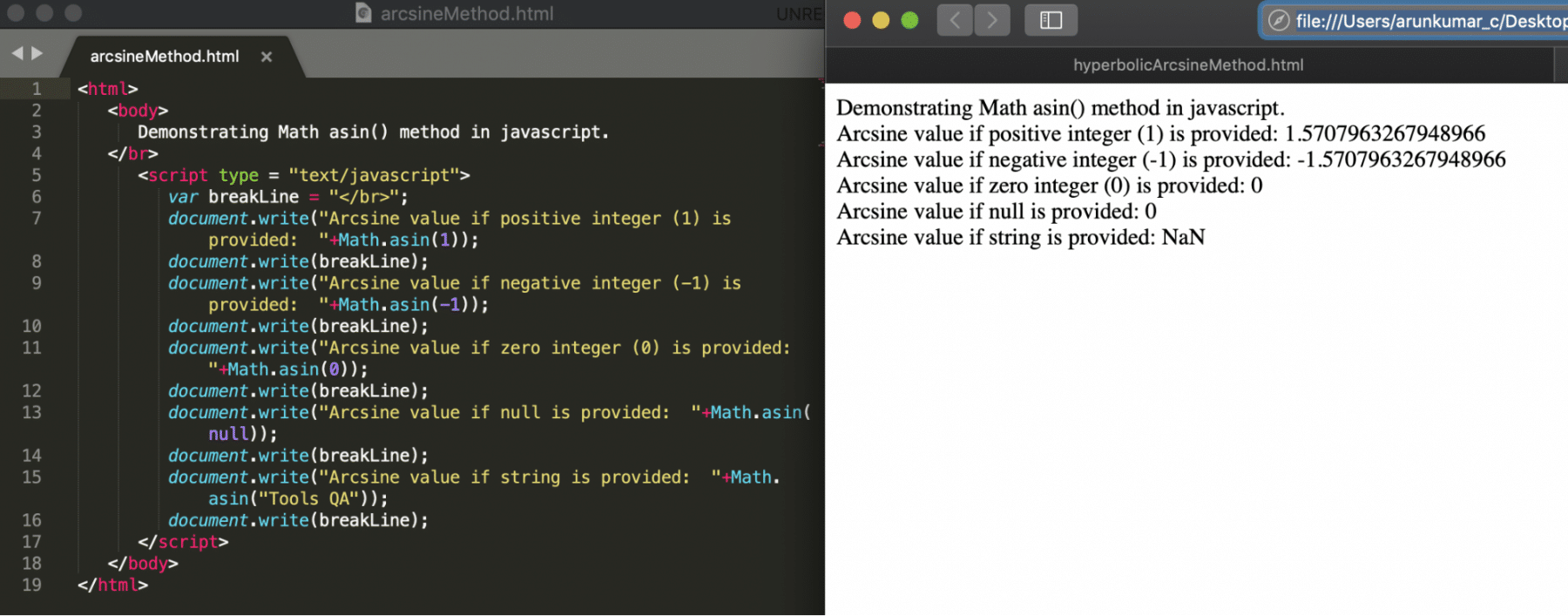#### asinh()

This function calculates the hyperbolic arcsine value for the provided input. Another name for asinh() is the "inverse hyperbolic sine" function. Moreover, its syntax looks like below:

Syntax:

``````var radians = -1;

``````

Let's understand the usage of asinh() for various variable types with the help of following code snippet:

``````<html>

<body>

Demonstrating Math asinh() method in javascript.

</br>

<script type = "text/javascript">

var breakLine = "</br>";

document.write("Arcsine value if positive integer (20) is provided:  "+Math.asinh(20));

document.write(breakLine);

document.write("Arcsine value if negative integer (-20) is provided:  "+Math.asinh(-20));

document.write(breakLine);

document.write("Arcsine value if zero integer (0) is provided:  "+Math.asinh(0));

document.write(breakLine);

document.write("Arcsine value if null is provided:  "+Math.asinh(null));

document.write(breakLine);

document.write("Arcsine value if string is provided:  "+Math.asinh("Tools QA"));

document.write(breakLine);

</script>

</body>

</html>

``````

Save the file with name hyperbolicArcsineMethod.html. After that, open it in any browser (Chrome, Firefox, or IE). It should show the output as: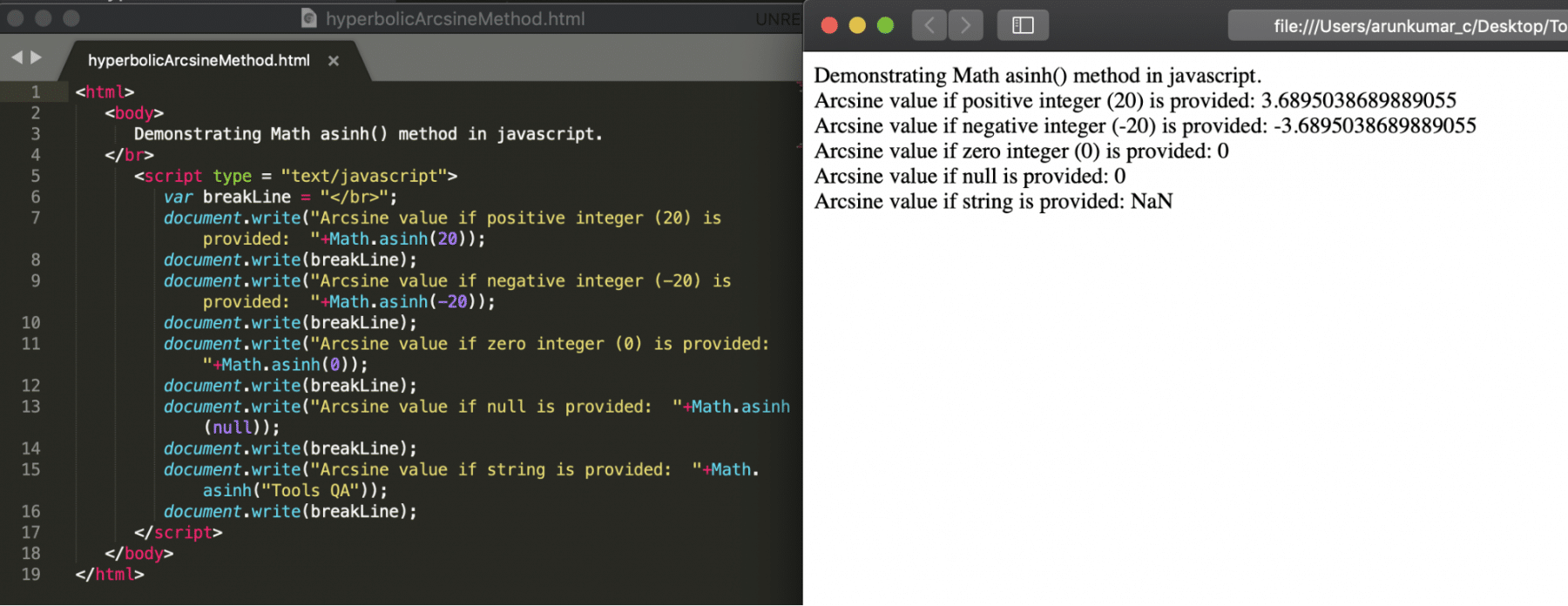#### Atan()

The atan() method returns the tangent value between -PI/2 to  PI/2. The atan() is nothing but arctangent(in radians) of number. As we know in mathematics,arctangent is an inverse of tangent. Additionally, its syntax looks like below:

Syntax:

``````var radians = -1;

``````

Let's understand the usage of atan() for various variable types with the help of following code snippet:

``````<html>

<body>

Demonstrating Math atan() method in javascript.

</br>

<script type = "text/javascript">

var breakLine = "</br>";

document.write("Arctangent value if positive integer (1) is provided:  "+Math.atan(1));

document.write(breakLine);

document.write("Arctangent value if negative integer (-1) is provided:  "+Math.atan(-1));

document.write(breakLine);

document.write("Arctangent value if zero integer (0) is provided:  "+Math.atan(0));

document.write(breakLine);

document.write("Arctangent value if null is provided:  "+Math.atan(null));

document.write(breakLine);

document.write("Arctangent value if string is provided:  "+Math.atan("Tools QA"));

document.write(breakLine);

</script>

</body>

</html>

``````

Save the file with name arctanMethod.html. After that, open it in any browser (Chrome, Firefox, or IE). It should show the output as: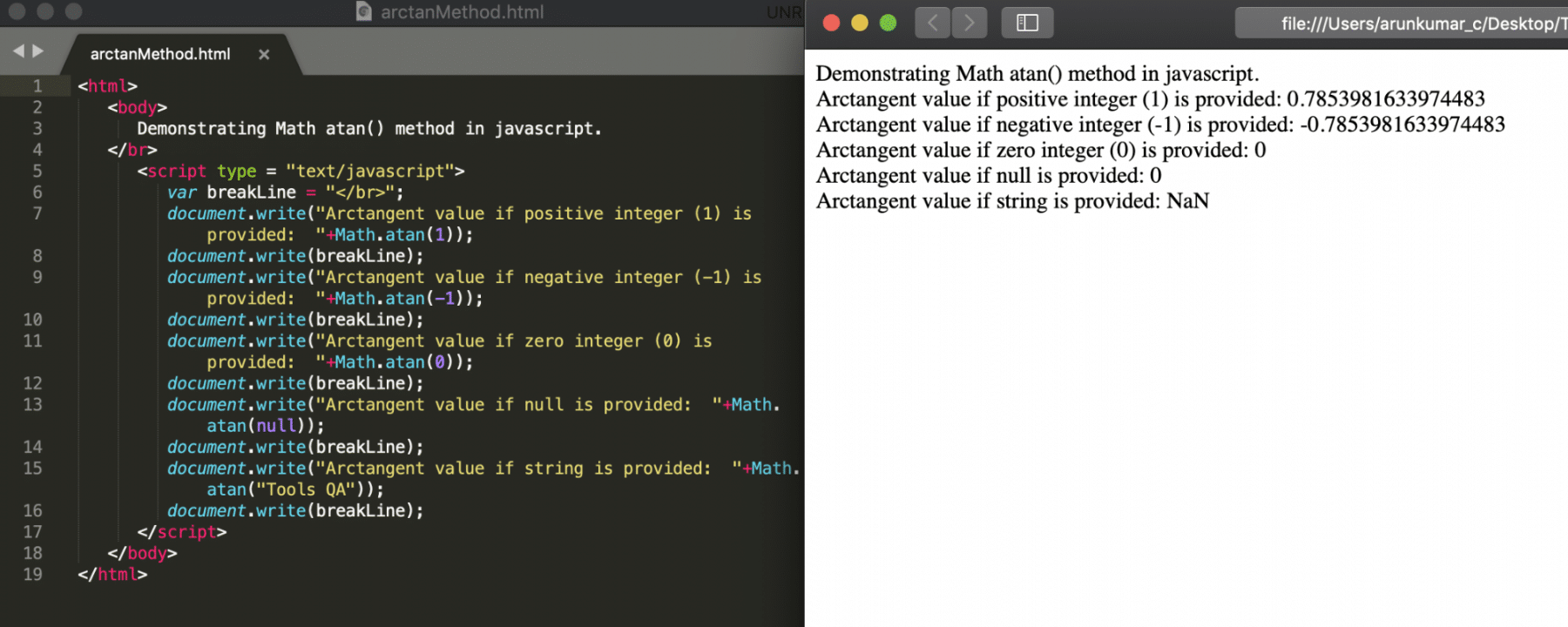#### Atanh()

The atanh() function returns the hyperbolic arctan value for the provided input. Another name for atanh() is the "inverse hyperbolic tangent" function. Additionally, its syntax looks like below:

Syntax:

``````var radians = -1;

``````

Let's understand the usage of atanh() for various variable types with the help of following code snippet:

``````<html>

<body>

Demonstrating Math atanh() method in javascript.

</br>

<script type = "text/javascript">

var breakLine = "</br>";

document.write("Arctangent value if positive integer (0.5) is provided:  "+Math.atanh(0.5));

document.write(breakLine);

document.write("Arctangent value if negative integer (-0.5) is provided:  "+Math.atanh(-0.5));

document.write(breakLine);

document.write("Arctangent value if zero integer (0) is provided:  "+Math.atanh(0));

document.write(breakLine);

document.write("Arctangent value if null is provided:  "+Math.atanh(null));

document.write(breakLine);

document.write("Arctangent value if string is provided:  "+Math.atanh("Tools QA"));

document.write(breakLine);

</script>

</body>

</html>
``````

Save the file with name hyperbolicArctanMethod.html and open it in any browser (Chrome, Firefox, or IE). It should show the output as: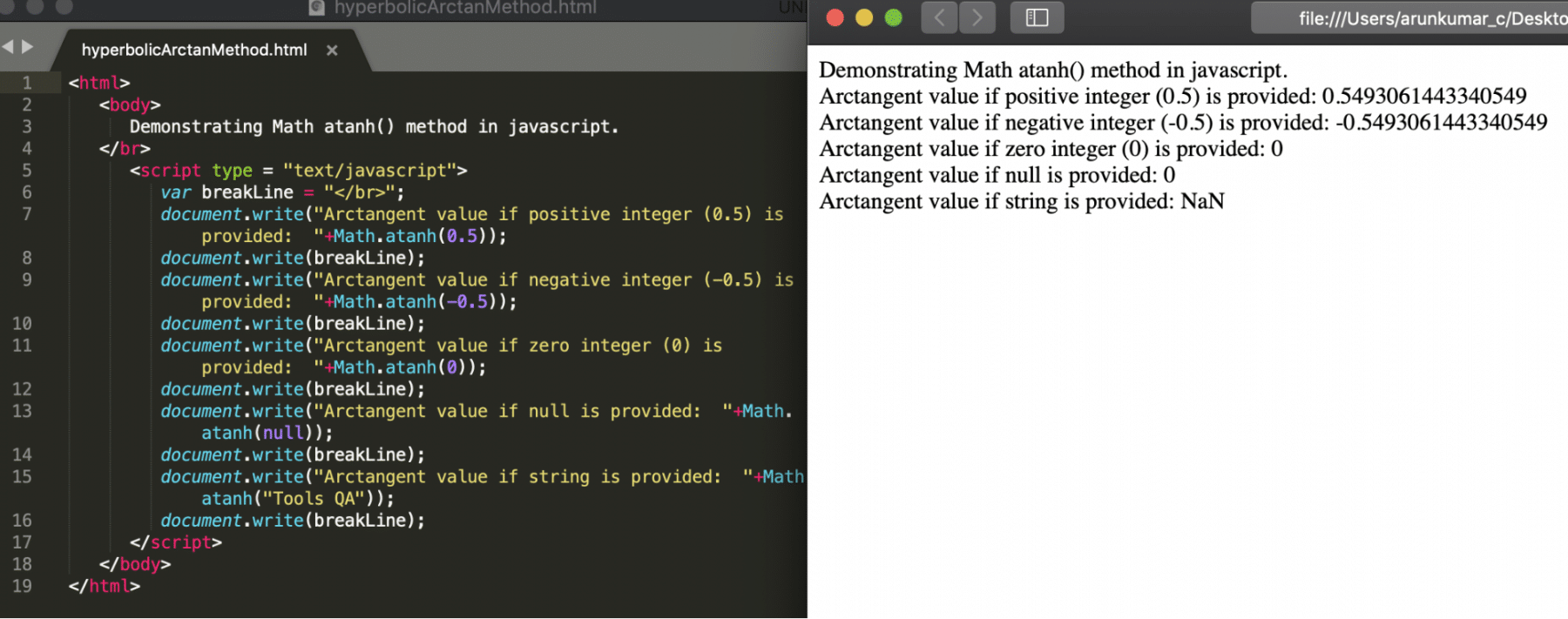#### Cbrt:

This function returns the cube root of the provided input. Its syntax looks like below:

Syntax:

``````var output = Math.cbrt(value);

``````

Let's understand the usage of cbrt() for various variable types with the help of following code snippet:

``````<html>

<body>

Demonstrating Math cbrt() method in javascript.

</br>

<script type = "text/javascript">

var breakLine = "</br>";

document.write("Cube root value if positive integer (27) is provided:  "+Math.cbrt(27));

document.write(breakLine);

document.write("Cube root value if negative integer (-27) is provided:  "+Math.cbrt(-27));

document.write(breakLine);

document.write("Cube root value if null is provided:  "+Math.cbrt(null));

document.write(breakLine);

document.write("Cube root value if string is provided:  "+Math.cbrt("Tools QA"));

document.write(breakLine);

document.write("Cube root value if decimal value (-5.5) is provided:  "+Math.cbrt(-5.5));

document.write(breakLine);

</script>

</body>

</html>

``````

Save the file with name cuberoot.html and open it in any browser (Chrome, Firefox, or IE). It should show the output as: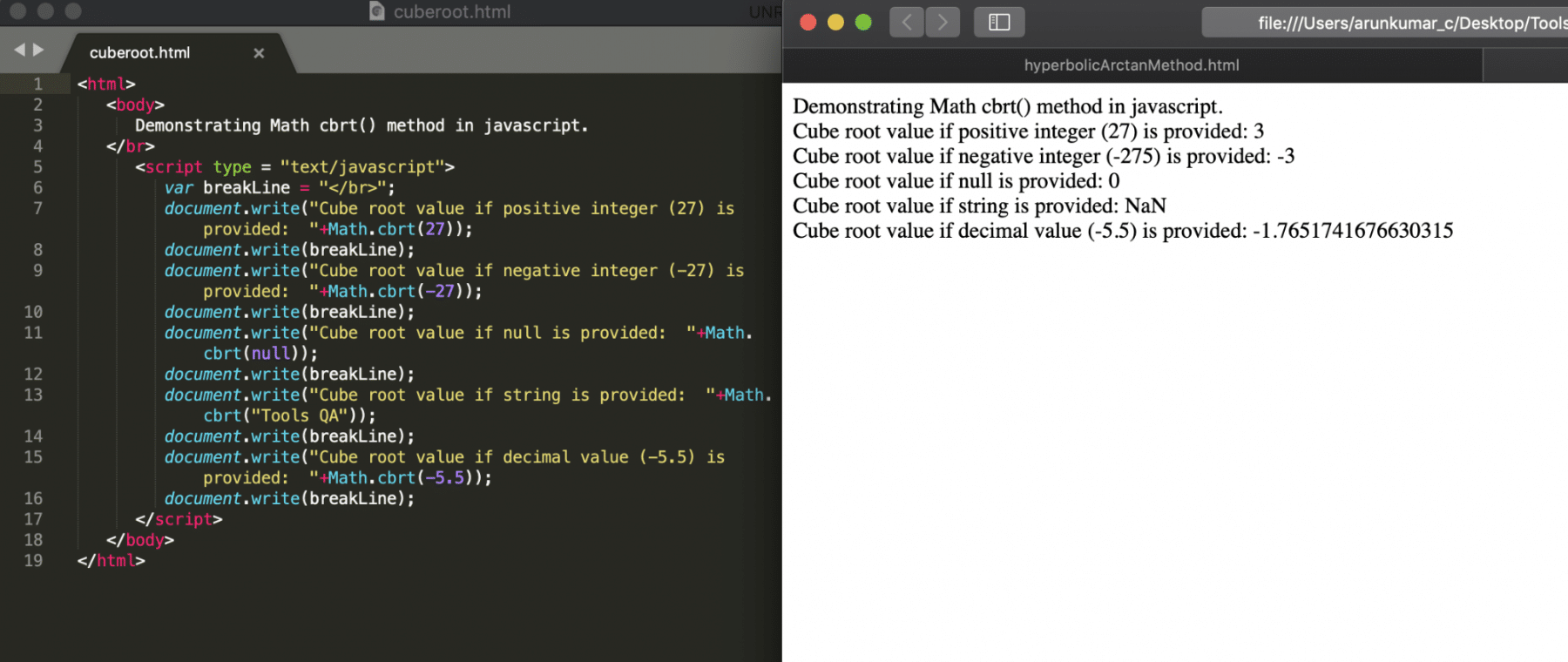#### sqrt()

This function returns the square root of the provided input. Its syntax looks like below:

Syntax:

``````var output = Math.sqrt(value);
``````

Let's understand the usage of sqrt() for various variable types with the help of following code snippet:

``````<html>

<body>

Demonstrating Math sqrt() method in javascript.

</br>

<script type = "text/javascript">

var breakLine = "</br>";

document.write("Square root value if positive integer (36) is provided:  "+Math.sqrt(36));

document.write(breakLine);

document.write("Square root value if negative integer (-36) is provided:  "+Math.sqrt(-36));

document.write(breakLine);

document.write("Square root value if null is provided:  "+Math.sqrt(null));

document.write(breakLine);

document.write("Square root value if string is provided:  "+Math.sqrt("Tools QA"));

document.write(breakLine);

document.write("Square root value if decimal value (-5.5) is provided:  "+Math.sqrt(-5.5));

document.write(breakLine);

</script>

</body>

</html>
``````

Save the file with name squareroot.html and open it in any browser (Chrome, Firefox, or IE). It should show the output as: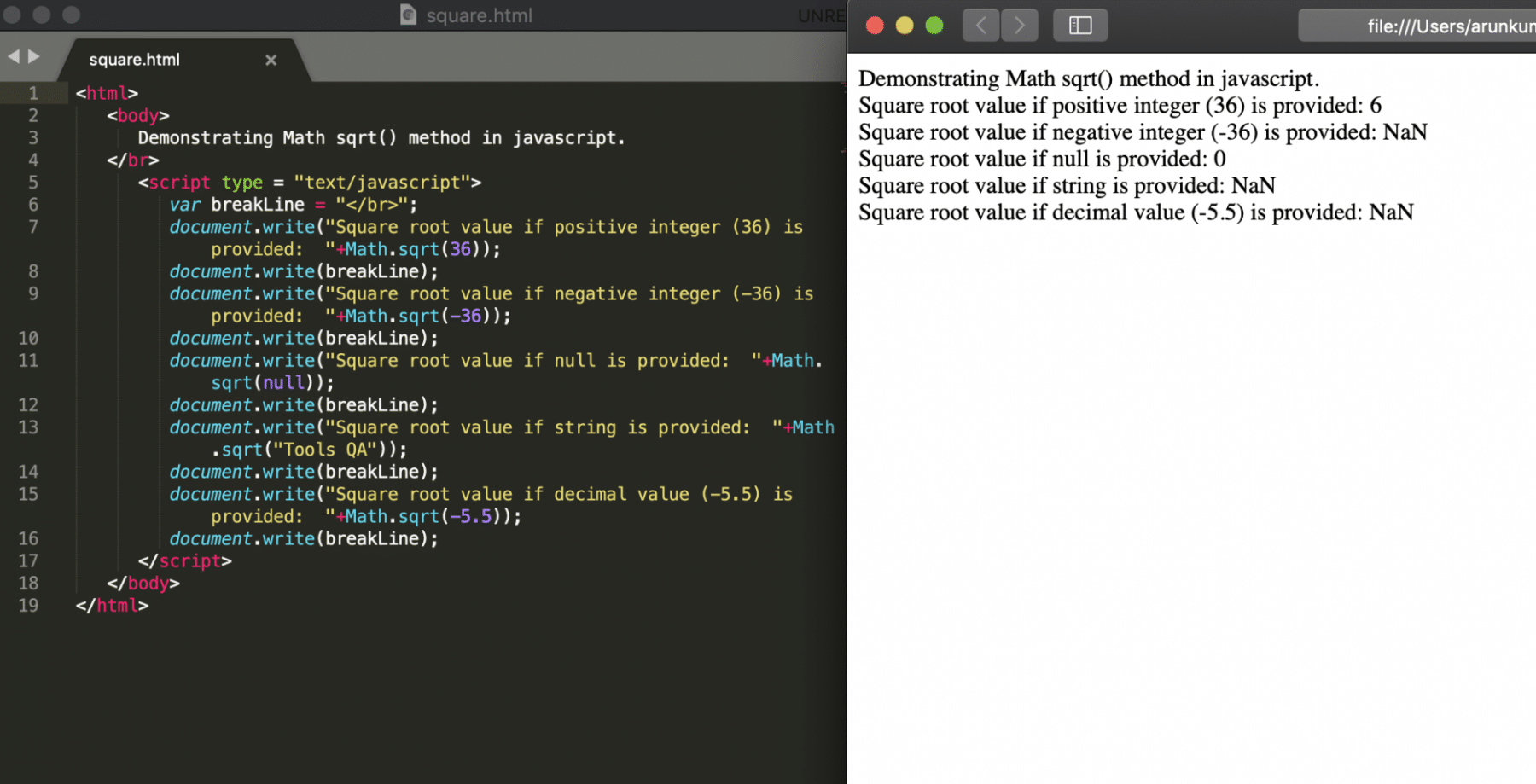#### ceil()

This function returns the ceiling value of the input. E.g., if the input is 5.3, then the ceiling value will 6. Its syntax looks like below:

Syntax:

``````var output = Math.ceil(value);
``````

Let's understand the usage of ceil() for various variable types with the help of following code snippet:

``````<html>

<body>

Demonstrating Math ceil() method in javascript.

</br>

<script type = "text/javascript">

var breakLine = "</br>";

document.write("Ceiling value if positive input value (5.3) is provided:  "+Math.ceil(5.3));

document.write(breakLine);

document.write("Ceiling value if negative input value (-5.8) is provided:  "+Math.ceil(-5.8));

document.write(breakLine);

document.write("Ceiling value if null is provided:  "+Math.ceil(null));

document.write(breakLine);

document.write("Ceiling value if string is provided:  "+Math.ceil("Tools QA"));

document.write(breakLine);

</script>

</body>

</html>

``````

Save the file with name ceil.html and open it in any browser (Chrome, Firefox, or IE). It should show the output as: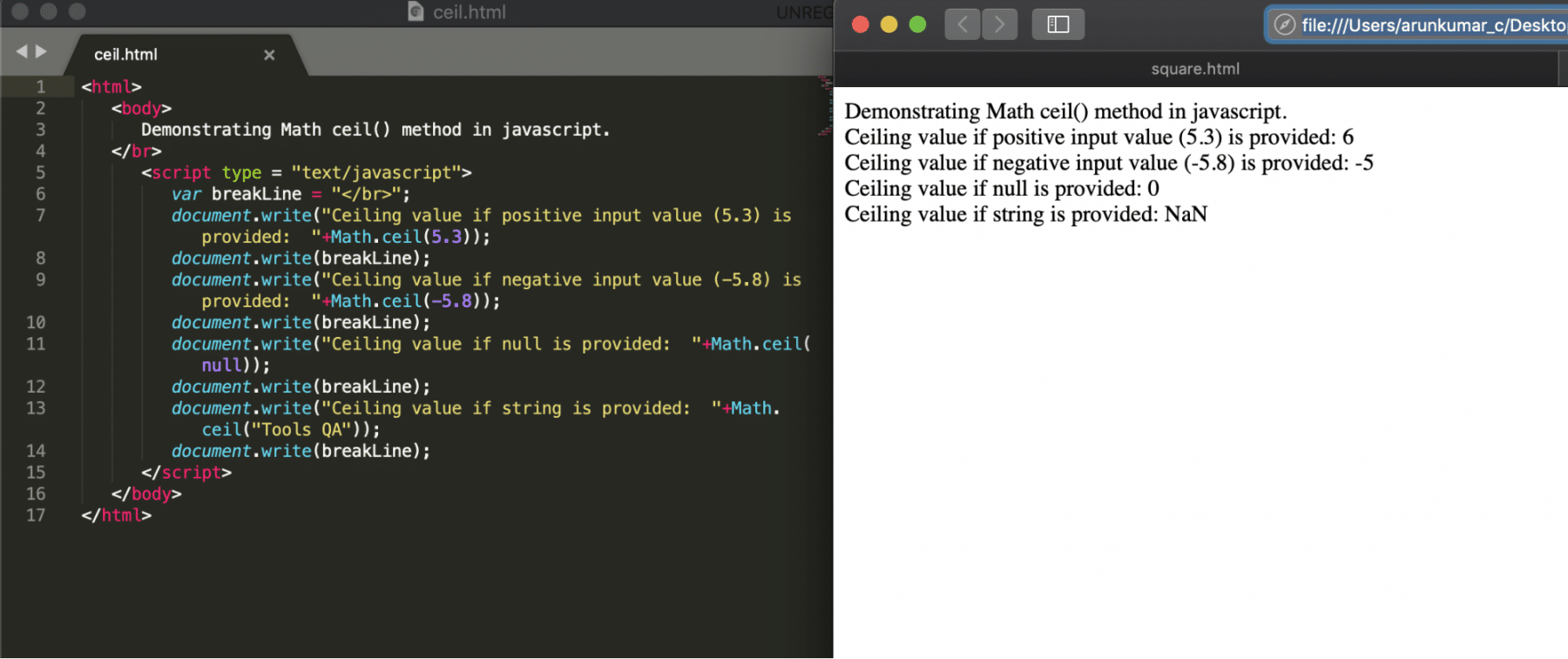#### Floor:

This function returns the floor value of the provided input. E.g., the floor value of 5.8 will be 5. Its syntax looks like below:

Syntax:

``````var output = Math.floor(value);
``````

Let's understand the usage of floor() for various variable types with the help of following code snippet:

``````<html>

<body>

Demonstrating Math floor() method in javascript

</br>

<script type = "text/javascript">

var breakLine = "</br>";

document.write("Floor value if positive input value (5.3) is provided:  "+Math.floor(5.3));

document.write(breakLine);

document.write("Floor value if negative input value (-5.8) is provided:  "+Math.floor(-5.8));

document.write(breakLine);

document.write("Floor value if null is provided:  "+Math.floor(null));

document.write(breakLine);

document.write("Floor value if string is provided:  "+Math.floor("Tools QA"));

document.write(breakLine);

</script>

</body>

</html>

``````

Save the file with name floor.html and open it in any browser (Chrome, Firefox, or IE). It should show the output as: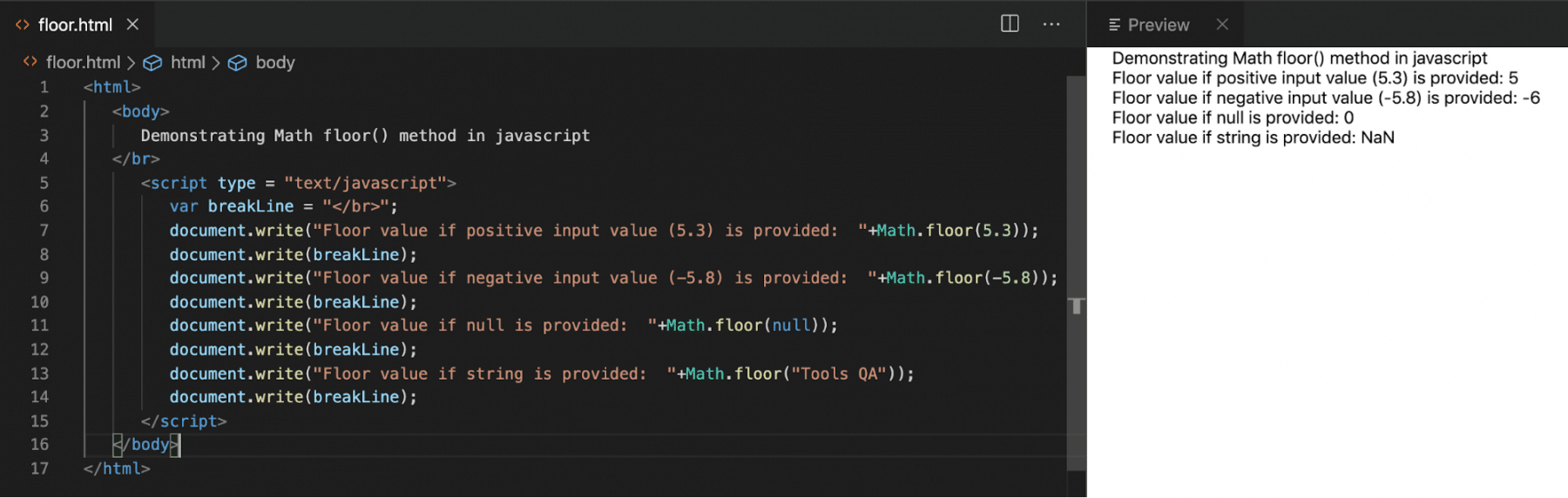#### Exp

This function returns the exponential value of the given input. Its syntax looks like below:

Syntax:

``````var output = Math.exp(value);
``````

Let's understand the usage of exp() for various variable types with the help of following code snippet:

``````<html>

<body>

Demonstrating Math exp() method in javascript

</br>

<script type = "text/javascript">

var breakLine = "</br>";

document.write("Exponential value if positive input value (5.3) is provided:  "+Math.exp(5.3));

document.write(breakLine);

document.write("Exponential value if negative input value (-5.8) is provided:  "+Math.exp(-5.8));

document.write(breakLine);

document.write("Exponential value if null is provided:  "+Math.exp(null));

document.write(breakLine);

document.write("Exponential value if string is provided:  "+Math.exp("Tools QA"));

document.write(breakLine);

</script>

</body>

</html>

``````

Save the file with name exp.html and open it in any browser (Chrome, Firefox, or IE). It should show the output as: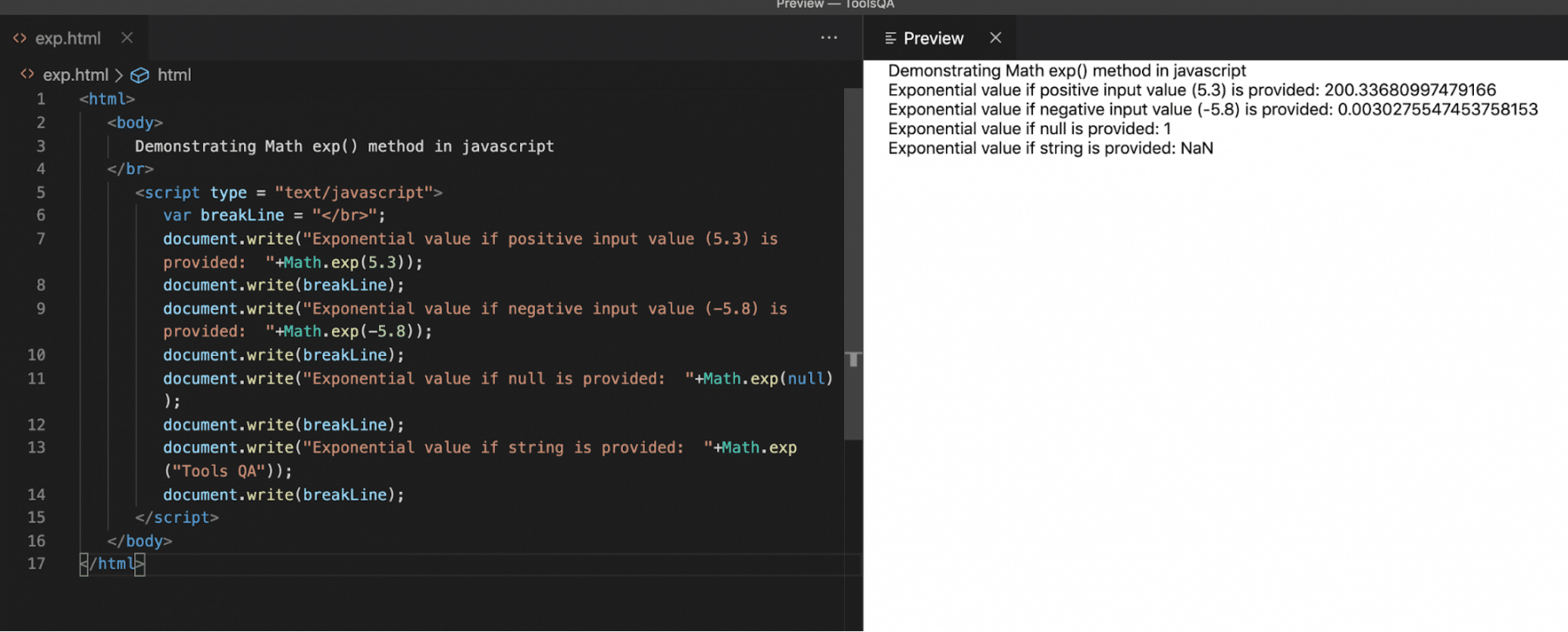#### Round:

This function returns the rounded value of decimals. Its syntax looks like below:

Syntax:

``````var output = Math.round(value);
``````

Let's understand the usage of round() for various variable types with the help of following code snippet:

``````<html>

<body>

Demonstrating Math round() method in javascript

</br>

<script type = "text/javascript">

var breakLine = "</br>";

document.write("Rounded value if positive input value (5.3) is provided:  "+Math.round(5.3));

document.write(breakLine);

document.write("Rounded value if negative input value (-5.8) is provided:  "+Math.round(-5.8));

document.write(breakLine);

document.write("Rounded value if null is provided:  "+Math.round(null));

document.write(breakLine);

document.write("Rounded value if string is provided:  "+Math.round("Tools QA"));

document.write(breakLine);

</script>

</body>

</html>

``````

Save the file with name round.html and open it in any browser (Chrome, Firefox, or IE). It should show the output as: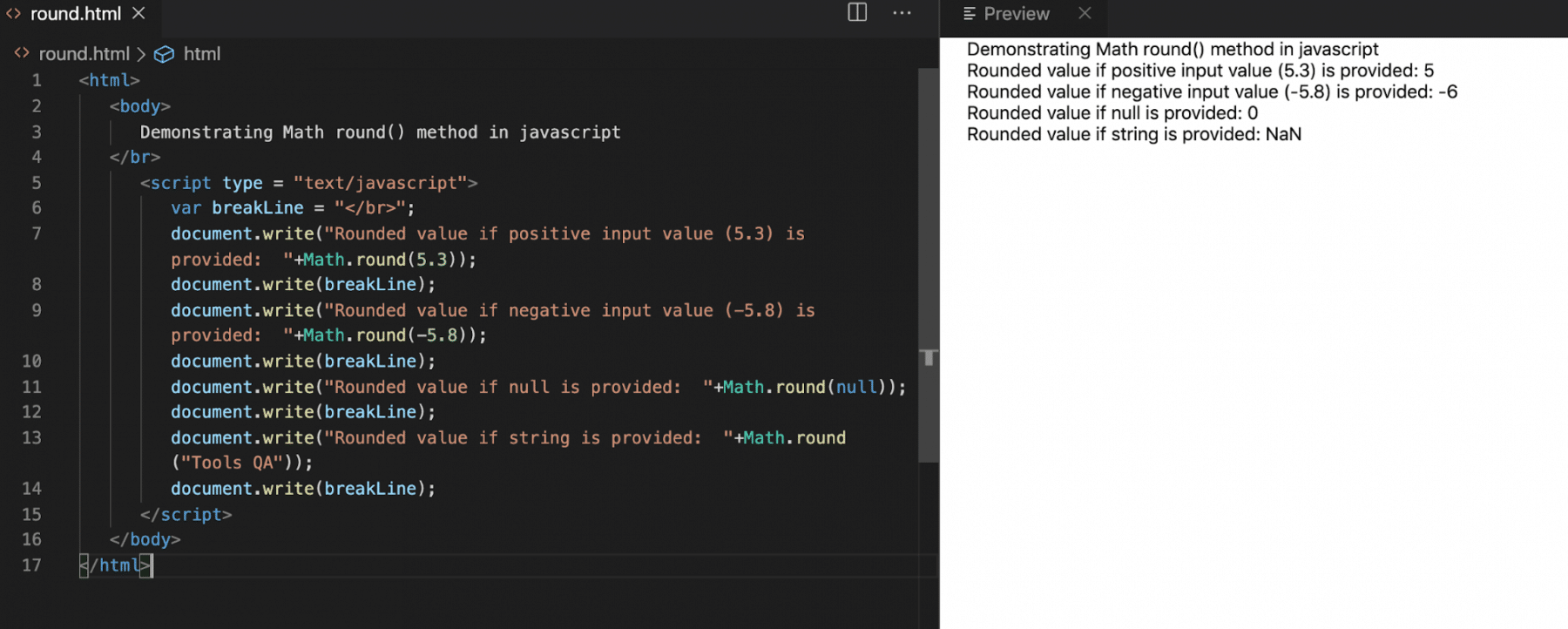#### Max

This function returns the maximum value in the given set of values. Its syntax looks like below:

Syntax:

``````var output = Math.max(v1,v2,v3,v4…..,vN);
``````

Let's understand the usage of max() for various variable types with the help of following code snippet:

``````<html>

<body>

Demonstrating Math max() method in javascript

</br>

<script type = "text/javascript">

var breakLine = "</br>";

document.write("Max value if positive input value (5.3,6.5,3.2,5.5,9,10.2) is provided:  "+Math.max(5.3,6.5,3.2,5.5,9,10.2));

document.write(breakLine);

document.write("Max value if negative input value (-5.8,-5.5,-4,-10,-3) is provided:  "+Math.max(-5.8,-5.5,-4,-10,-3));

document.write(breakLine);

document.write("Max value if (-1,-2,-4,-6,null) is provided:  "+Math.max(-1,-2,-4,-6,null));

document.write(breakLine);

document.write("Max value if string is provided:  "+Math.max("Tools QA","test","Another"));

document.write(breakLine);

</script>

</body>

</html>
``````

Save the file with name max.html and open it in any (Chrome, Firefox, or IE)browser It should show the output as: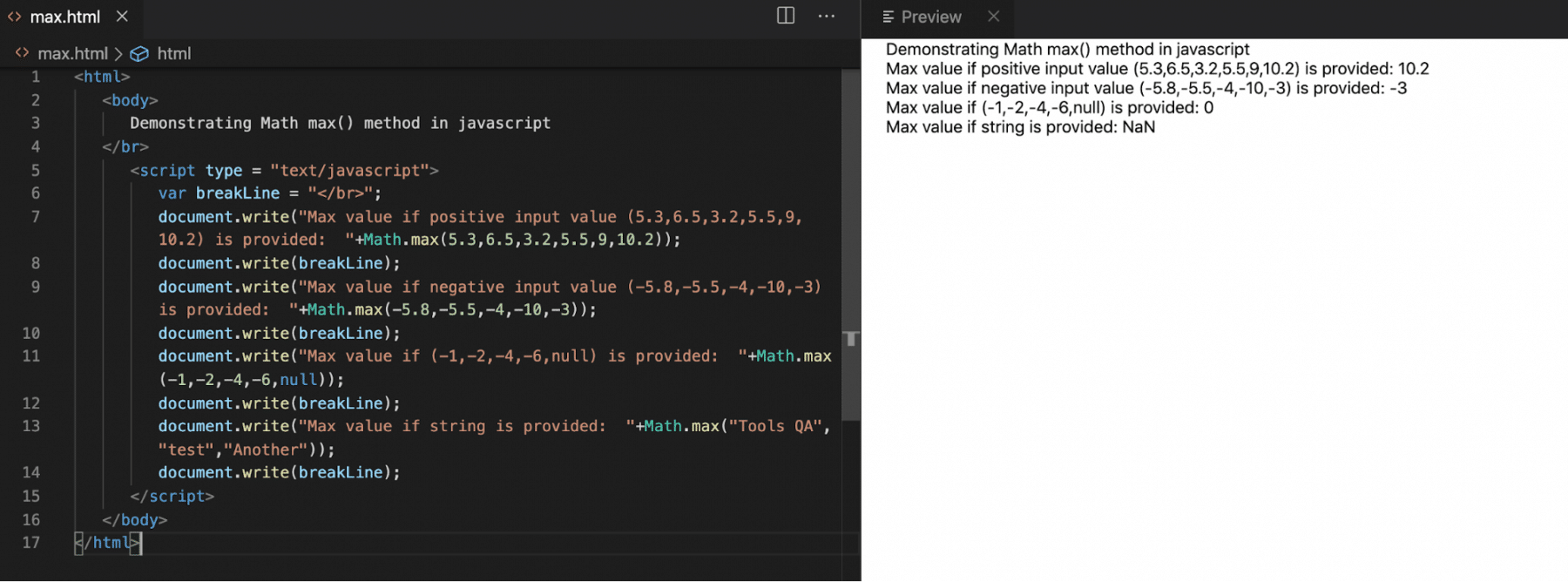#### Min

This function returns the minimum value in the given set of values. Its syntax looks like below:

Syntax:

``````var output = Math.min(v1,v2,v3….,vN);
``````

Let's understand the usage of min() for various variable types with the help of following code snippet:

``````<html>

<body>

Demonstrating Math min() method in javascript

</br>

<script type = "text/javascript">

var breakLine = "</br>";

document.write("Min value if positive input value (5.3,6.5,3.2,5.5,9,10.2) is provided:  "+Math.min(5.3,6.5,3.2,5.5,9,10.2));

document.write(breakLine);

document.write("Min value if negative input value (-5.8,-5.5,-4,-10,-3) is provided:  "+Math.min(-5.8,-5.5,-4,-10,-3));

document.write(breakLine);

document.write("Min value if (-1,-2,-4,-6,null) is provided:  "+Math.min(-1,-2,-4,-6,null));

document.write(breakLine);

document.write("Min value if string is provided:  "+Math.min("Tools QA","test","Another"));

document.write(breakLine);

</script>

</body>

</html>
``````

Save the file with name min.html and open it in any browser (Chrome, Firefox, or IE). It should show the output as: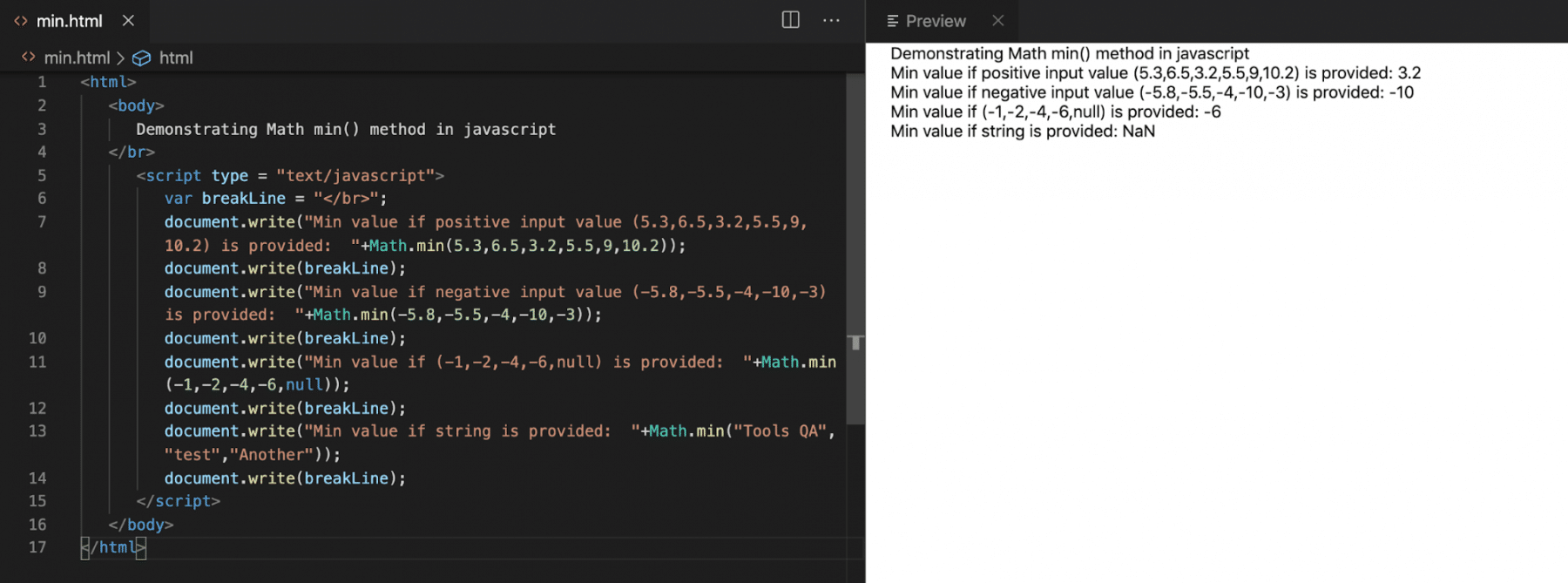#### Pow

This function returns the power value of the given input. Its syntax looks like below:

Syntax:

``````var output = Math.pow(value, toPower);
``````

Let's understand the usage of pow() for various variable types with the help of following code snippet:

``````<html>

<body>

Demonstrating Math pow() method in javascript

</br>

<script type = "text/javascript">

var breakLine = "</br>";

document.write("2 to the power of 3 is :  "+Math.pow(2,3));

document.write(breakLine);

document.write("2 to the power of -3 is  "+Math.pow(2,-3));

document.write(breakLine);

document.write("5.5 to the power of 4 is  "+Math.pow(5.5,4));

document.write(breakLine);

document.write("Tools QA to the power of 3 is  "+Math.pow("Tools QA",3));

document.write(breakLine);

</script>

</body>

</html>

``````

Save the file with name pow.html and open it in any browser (Chrome, Firefox, or IE). It should show the output as: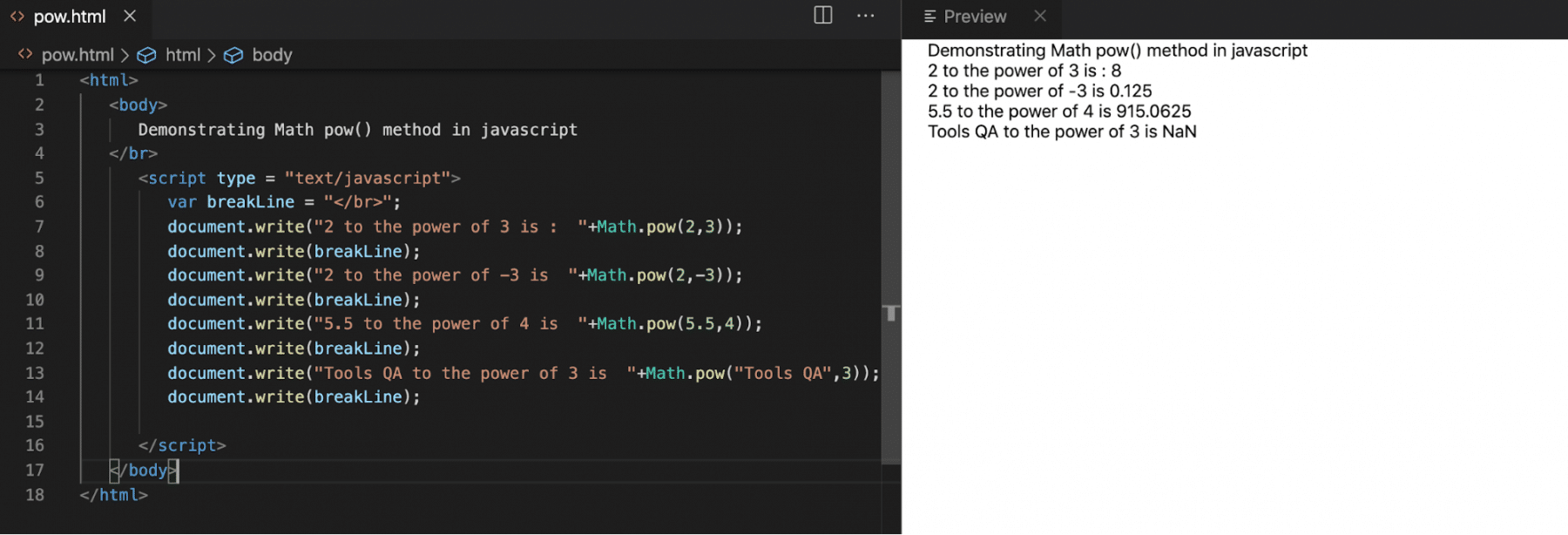#### Random

This function returns a random value between 0 to 1. Its syntax looks like below:

Syntax:

``````var output = Math.random();
``````

Let's understand the usage of random() for various variable types with the help of following code snippet:

``````<html>

<body>

Demonstrating Math random() method in javascript

</br>

<script type = "text/javascript">

var breakLine = "</br>";

document.write("1st Random value is :"+Math.random());

document.write(breakLine);

document.write("2nd Random value is :"+Math.random());

document.write(breakLine);

document.write("3rd Random value is :"+Math.random());

document.write(breakLine);

document.write("4th Random value is :"+Math.random());

document.write(breakLine);

document.write("5th Random value is :"+Math.random());

document.write(breakLine);

</script>

</body>

</html>
``````

Save the file with name random.html and open it in any browser (Chrome, Firefox, or IE). It should show the output as: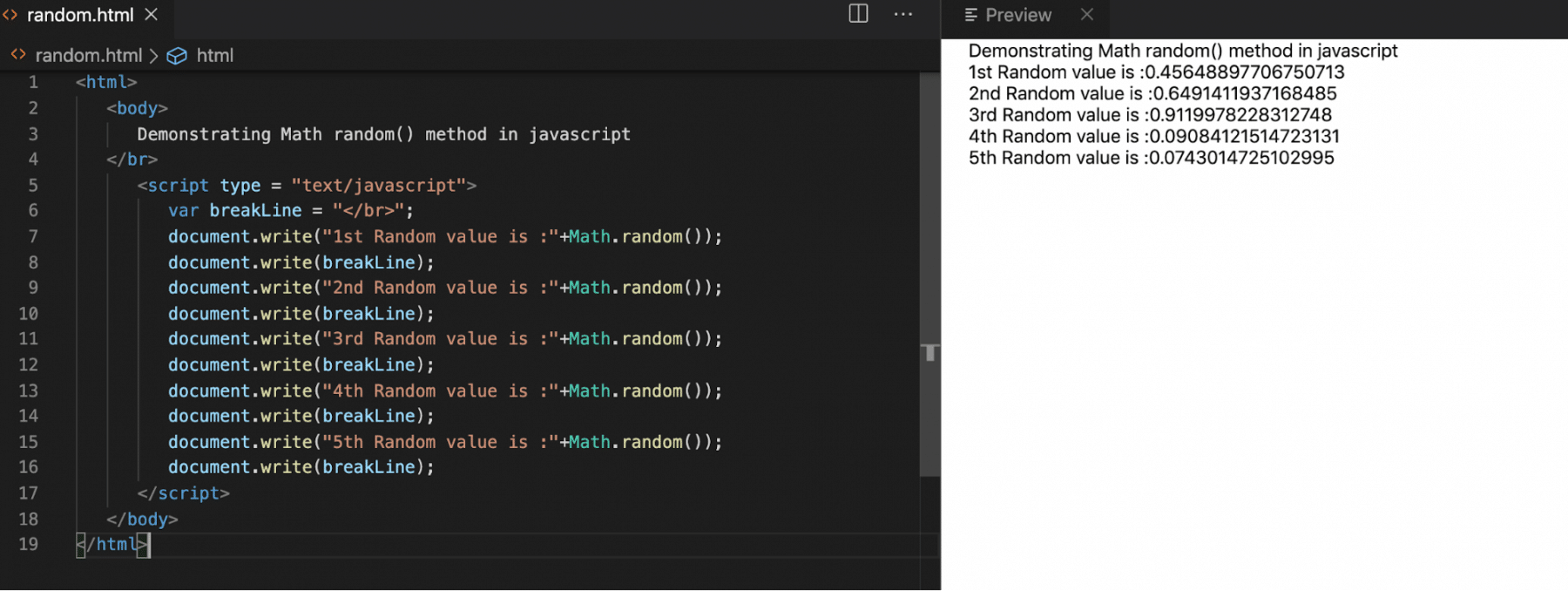## Key Takeaways

• The "Math" object provides various properties and methods which can perform mathematical operations in JavaScript.
• Moreover, you can use mathematical constants such as PI, Log values, etc. using the properties provided by Math object such as  Math.PI, Math.Log2E.
• In addition to the above, you can invoke the methods provided by Math object such as abs(), asin(), ceil(), etc. to perform various mathematical operations on multiple variables.

To conclude, let's now move to the next article, "Deep dive into objects," to understand the concepts of Objects in JavaScript in more detail.